## Friday, 3 August 2012

### An open problem, matlab and randomness

Just sharing a question that I've done recently, and that actually recalls something that I intend to write some times before but gave up very soon.

Now consider a hypothetical cat and a mouse on a circle chasing each other.
1) Cat's speed is random from -2 to 2 (-ve = anti-clockwise)
2) Mouse's speed is i) fixed at 1 ii) random within a certain range.
3) The cat "catches" the mouse if the cat "catches" the mouse or the mouse "catches" the cat.
4) The mouse starts at random position of circle (radius 2) while cat starts at x=0.

And let's deal with the matlab code...
-------------
clear
vm=zeros(401,1000)+1; %vm = speed of mouse, we are generating a matrix here in case we will use random speed later
d=rand(401,1000)*4*pi %random position of mouse
A=zeros(401,1000);
for I=1:401
vc=(I-201)/100;
for J=1:1000
if vc > vm(I,J)
A(I,J)=abs(vc*d(I,J)/(vc-vm(I,J));
else
A(I,J)=abs(vc*(4*pi-d(I,J))/(vc-vm(I,J));
end
end
end
for I=1:201
y(I)=(mean(A(200+I,:))+mean(A(202-I,:)))/2;
end
%direction of cat is the not our concern (though it behaves differently)
y(101)=(y(100)+y(102))/2;
%If vc=vm the cat never catches the mouse and by default distance travelled = 0 is not our interest, so delete that entry and smooth the curve.
x=0:0.01:2;
plot(x,y)
----------
Output:

(In fact I'm not using y(101) as the mean of y(100) and y(102) here because it's supposed to tend to infinity and hence it's not the mean of the neighbouring terms.)

What can you observe? A significant peak like the IR spectrometry or something else? Even though we are generating randomness, this is pretty much an "expected" result --- let see what we can interpret here.

1) Formula: distance = speed * position diff / speed diff.
In our very first model, position of mouse is the only random variable, and therefore the distance is approximately inversely proportional to the inverse of the speed difference (vc - 1), and hence it gives the above graph.

2) Along the speed
As you may noticed, the graph is not symmetrical but this can be accounted easily. When vc tends to zero by the above formula the distance tends to zero (as the rest of the terms are finite). It's also somehow "common sense" as the cat just doens't move and wait the mouse to catch the cat. At the same time when the speed tends to infinity the distance travelled tends to the positional difference between the cat and the mouse because the cat catches the mouse when the mouse hasn't move a lot. As a result it has a non-zero asymptote.

3) Clockwise vs anti-clockwise
The direction of the cat does not change the nature of distance travelled vs speed of cat. However we should notice that with negative speed (anti-clockwise) the distance travelled is very short because the cat and the mouse are approaching each other.

Now we can deal with the graph analytically.

4) Transient behavior from vc=0 to vc=-2
As discussed the behavior of vc= - 2 is similar to vc=2, i.e. it has the same asymptote at the negative end. That reminds us the hyperbolic function, and actually it is a hyperbolic function when you look at the formula. We can conclude that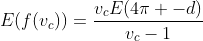for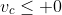. This is still a "distribution" concerning the randomness of positional difference d, but it can be easily calculated since it's a uniformly distributed probability function.

5) Closed form of the function.
At this point we are able to "separate" the negative part and positive part of velocity against distance travelled from the original graph in which merge both sets of data together. You might notice that at vc=0 the graph is not differentiable. However the formula didn't change as under the if conditions the formula for A is the same till vc = 1 (which is the fixed speed of the mouse), and with the abs() ahead you know that it's just the absolute value of the function above.

Lastly, we know that when vc > 1 the positional function reverts the positional difference from (4pi-d) to d. However it doesn't affect our calculation as for a uniform distribution P(x) from 0 to k, we have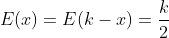, and therefore here we have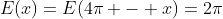. Therefore we have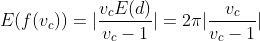.

In order to merge the positive and negative part we have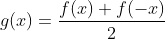, and finally we get the analytical expression for the above graph: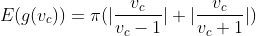which is identical to the graph above when the # of trials is large enough.

However, what will happen when we choose random vm? For example, in matlab code using vm=rand(401,1000)? Or vm = (rand(401,1000)*4)-2?

With simple plotting we can only observe complete randomness:

Completely random? Yes? No? We will deal with this question next time.